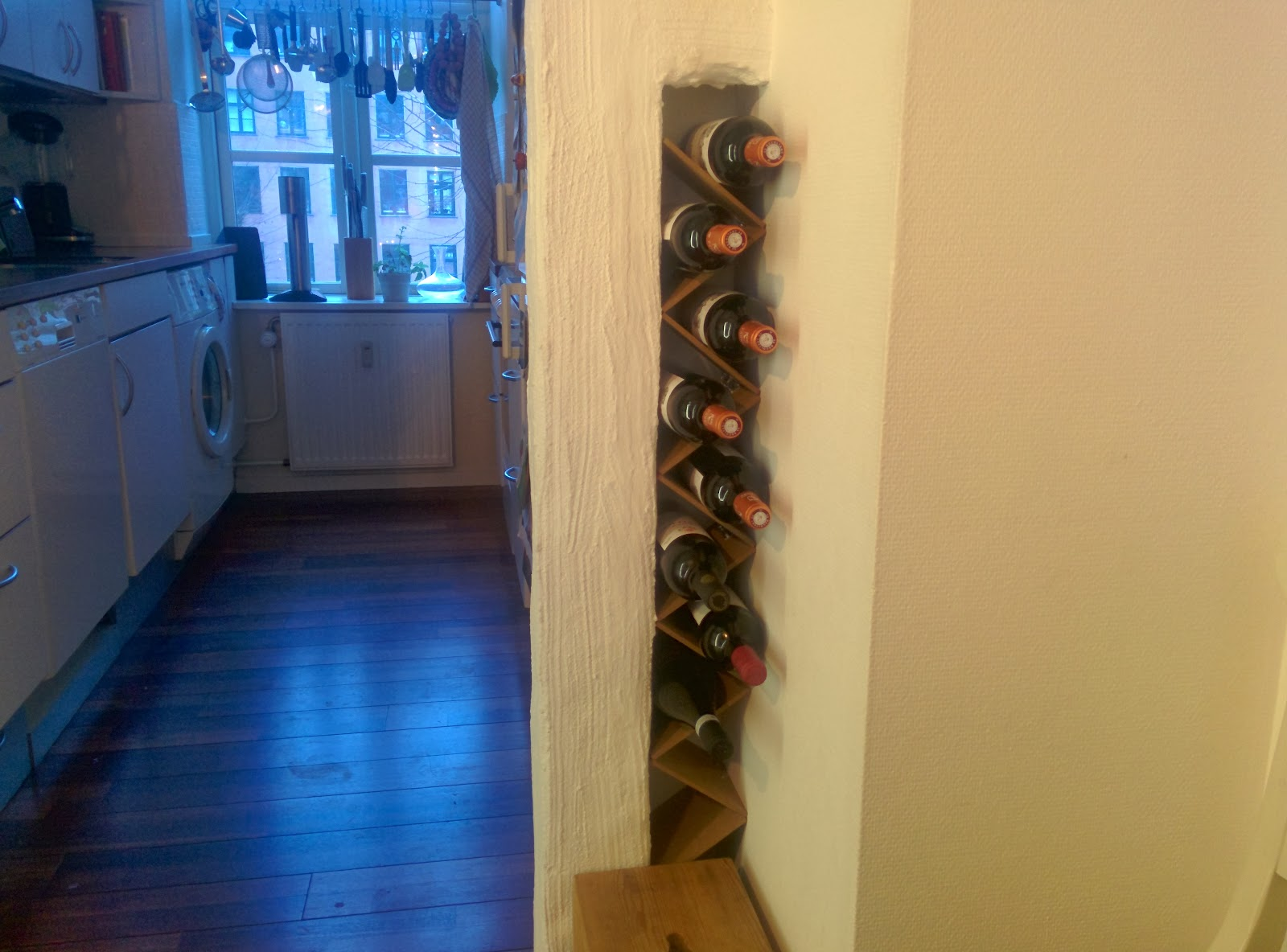## Sunday, January 26, 2014

### The math in buiding a wine rackBetween my kitchen and my dining room I have a 12 cm wide cavity. I thougth that this cavity was ideel for a wine rack. My plan was to clamp wooden boards together with hinges and let the tension hold them in place. The mathematician in me wanted to calculate how wide, w, the boards should be, and thougth it was reasonable to be able to place a bottle with diameter 9 in the rack (the bottles I had was no more than 7 cm in diameter). The math in this is that the wooden boards gave a equilateral triangle with high h = 12 cm and the radius r of the escribed circle should be 9/2 cm. This gives two right-angled triangles and Pythagoras gives us two equations:
• (h - r)2 = r2 + (w - x)2
• w2 = h2 + x2
Combining the two equations we have (h - r)2 = r2 + (w - (w2 - h2)1/2)2. By insetting r = 9/2 and h = 12 I used WolframAlpha to calculate the wide to be exact 15 cm. The only thing left to do is to buy the material and make the actually wine rack.The final result